The principle of mathematical induction

(notes by Roberto Bigoni)

Many mathematical theorems state that a property is true for every element of a definite set. If A is the set and a is an element of A and we use the logical symbolism, we can write: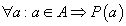The translation of this statement may be:"It is always true that, if a is a A, then a has the property P.

Most of these theorems can be demonstrated using the deductive method: starting from the hypothesis, recalling postulates and/or previously demonstrated theorems and applying general logical laws, one can reach the thesis.

Example: "(It is always true that) if τ is a triangle, then the sum of its internal angles equals the straight angle".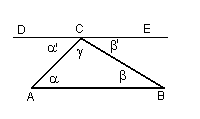Let ABC be a triangle and let α, β and γ be their internal angles. Let DE be the straight line parallel to AB through C (which exists and is unique: postulate). The angle DCA = α'=α because it is the internal alternate angle with respect to the angle ABC (theorem). The angle ECB = β'=β because it is the internal alternate angle with respect to the angle CBA (theorem). α'+γ+β' = DCE which is a straight angle. Sums of equal quantities are equal (general logical principle) so also α+β+γ is straight angle.

Mathematicians can also use proofs by contradiction: the falsity of the thesis would imply contradictory consequences, so the thesis must be true.

For example: "The prime numbers are infinite".

As is known, prime numbers are natural numbers greater than 1 and divisible only by themselves and 1.

If we suppose that the number of primes is finite, we must admit the existence of their maximum m and of the number p given by the product of all the primes from 2 to m: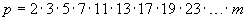We must also admit the existence of the natural number s immediately following p: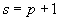This number s can't be prime, because it is greater than m, but if we divide it by any prime number from 2 to m, we get the remainder 1, so it is not divisible by any prime number less than or equal to m; then it should be divisible by some prime numbers greater than m, but our assumption is that these numbers do not exist.

Therefore, if we admit that the number of primes is finite, we get a contradictory conclusion; so we must admit that the number of primes is infinite.

In order to give the mathematics an axiomatic foundation, some mathematicians in the late 19th century, mainly J. W. R. Dedekind and G. Peano, found it necessary to admit a further way to demonstrate the trueness of the logical statements P(n) about the natural numbers n. This is the principle of mathematical induction.

Let f  be a map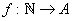, where N is the set of the natural numbers and A a predefined set. A sequence in A is the set of the elements of A which correspond in f  to the natural numbers.

The elements of a sequence may be written using the same symbol a together with the correspondig integer number. This number, the index, is smaller on the right: a1, a2, a3,....ai,...
ai is the i-th element of the sequence.
The whole sequence may be denoted in the following way: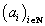.

For example, the sequence of the odd numbers (di)i ∈ N = {1,3,5,7,....} may be denoted by di=2i-1 where d1=1, d2=3, d3=5,...

A sequence of logical propositions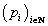is a sequence of statements with the same predicate referred to the elements of a sequence of natural numbers.

For example:

• pi = "(2i-1) is an odd number";
• qi = "(2i-1) is a prime number".

Intuitively we know that all the pi are true, and that not all the qi are true. But intuition is insufficient in mathematics. We need a formal procedure to validate a whole infinite set of statements. The mathematical induction principle is admitted as a formal procedure of validation.

 Given a sequence of logical propositions, if we find that: p1 is true; if pi is true, then pi+1 is true; we can say that all the pi are true.

EXAMPLES

1.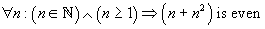that is: the sum of a natural number n ≥ 1 and its square n2 is an even number.

The statement may be easily demonstrated by deduction:

• if n is even, we have the sum of two even numbers;
• if n is odd, we have the sum of two odd numbers.

In both cases, the result is even.

Applying the mathematical induction principle, we may proceed in the following way:

•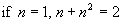, so the statement is true.
•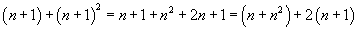that is, if we admit the trueness of the identity for a number n, the number n+1 is such that the sum between it and its successive number is a sum between two even numbers, so this sum is even.

• Since the property is true when n=1 and, if accepted for a number n, it is true when applied to the successive number n+1, therefore it is true for all the natural numbers.

2.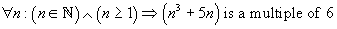that is: the sum of the cube of a natural number n ≥ 1 and the quintuple of the same number is a multiple of 6.

We can also in this case demonstrate the statement by deduction:

• the addends of the sum are either both even or both odd, so their sum is even; if this sum is also multiple of 3, it is multiple of 6;
• if n=1 or n=2, the sum is 6 or 18 and the statement is verified;
• if n≥3 we have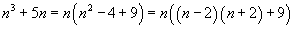where the statement is obvious when n is multiple of 3;
alternatively, either (n-2) or (n+2) is multiple of 3, thus their product is multiple of 3;
we can let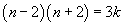so that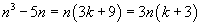evidencing that the sum is also multiple of 3.

Applying the mathematical induction principle, we may proceed in the following way:

•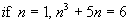; so the statement is true.
•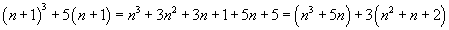• In the previous example we have seen that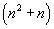is even, therefore also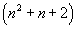is even and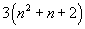is multiple of 6.
•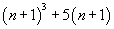is the sum of two multiples of 6, so it is multiple of 6.

• Since the statement is true when n=1 and, if it is true for any n then it is true for the successive number n+1, it is always true.

3.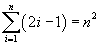that is: the sum of the first n odd natural numbers equals the square of n.

The statement is evidently true if n is 1.

If we admit that it is true for the sum of the first n odd numbers, can we demonstrate that it is true also if we extend the sum to the first n+1 odd numbers, that is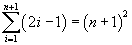?

Demonstration: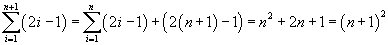4.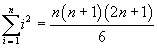that is: the sum of the squares of the first n natural numbers equals the sixth part of the product between n, its successive number and the successive number of its double.

This is immediate if n=1.

If we admit the trueness of the statement for the first n numbers, can we demonstrate that it is true also if we extend the sum to the first n+1 numbers, that is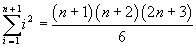?

Demonstration: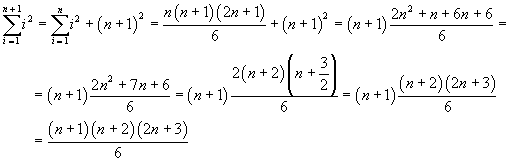5.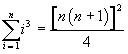that is: the sum of the cubes of the first n natural numbers equals the fourth part of the square of the product between n and its successive number.

This is immediate if n=1.

If we admit the trueness of the statement for the first n numbers, can we demonstrate that it is true also if we extend the sum to the first n+1 numbers, that is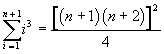?

Demonstration: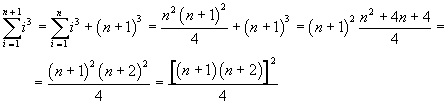This equality has the interesting variant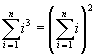that is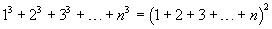In fact, in the equality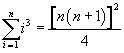the right side can be written as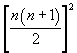and it's easy to demonstrate that the base of the square equals the sum of the first n natural numbers.

6.If n=1, the equality becomes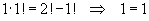.

If a number n verifies the equality, we must have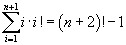Demonstration: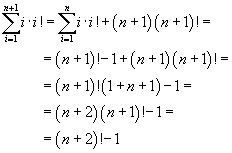7. Mengoli's series.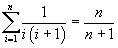The equality is immediate if n=1.

If we admit the equality for n we obtain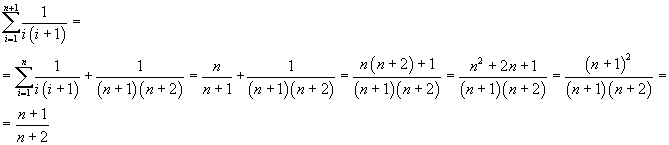so also n+1 verifies the identity, therefore it is generally true.

8. Geometric series.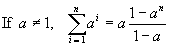The equality is immediate if n=1.

If we admit the equality for n we obtain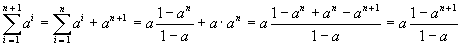so it holds also for n+1 and therefore it is generally true.

9.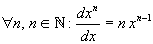that is: the derivative of the n-th power of the base x with respect to the base itself is given by the product between the exponent n and the n-1-th power of the base.

If n=1 the equality results true.

From the validity for n one can deduce the validity for n+1, that is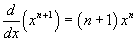Demonstration: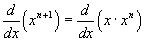The product rule of derivation of a product gives: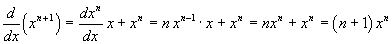10.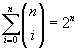This identity can be proved directly by applying the binomial theorem: infact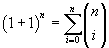It may be interesting, however, to prove this identity by induction.

We can immediately see that it is true if n=1.

For n+1 we have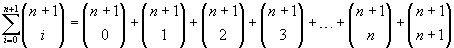Binomial coefficients are such that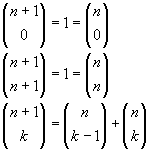so we can write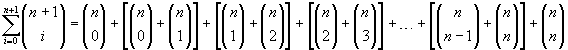that is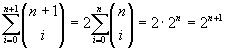11.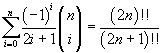where the double exclamation mark after a natural number indicates the double factorial of the number itself, that is, if the number is even, the product of all even numbers that precede it and, if the number is odd, the product of all odd numbers that precede it. Additionally -1!! = 0!! = 1

If n=0, both sides of the identity have value 1.

We must demonstrate that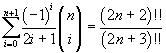If, in order to simplify the exposition, we assume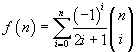we must demonstrate that, for every n>0,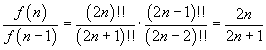For this purpose, we observe that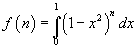and also, assuming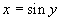,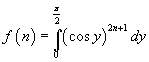In this integral the antiderivative of the integrand function can be evaluated by parts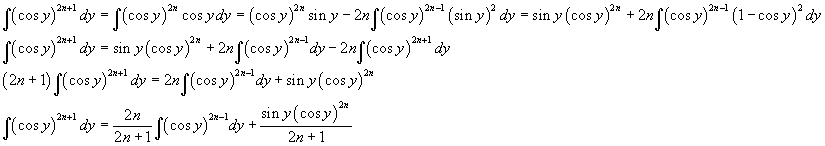Therefore the first fundamental theorem of calculus gives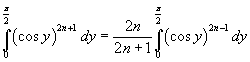The integral in the right side is f(n-1), so, ultimately,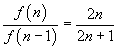12.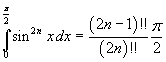When n=0, the left side is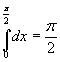The right side is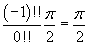So the identity (12) is true for n=0. Assuming (12) true for a given n we need to prove that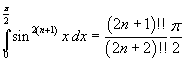The antiderivative of the integrand at the left side can be evaluated by parts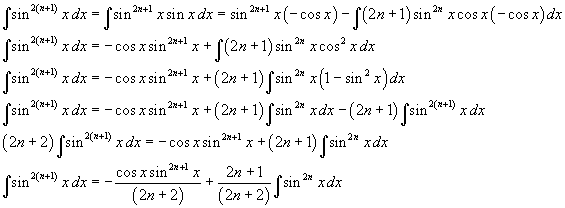The definite integral is therefore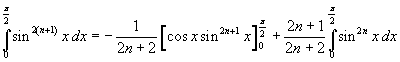Since the first addend in the right side is 0 and the integral in the second addend coincides with the left side in (12) we have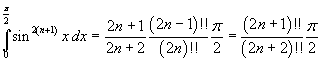Beware of rash conjectures

If, given a circumference, we consider 2 points on it, and we draw the chord between them, the circle is divided into 2 regions.If we consider 3 points and trace all the possible chords between them, the circle is divided into 4 regions.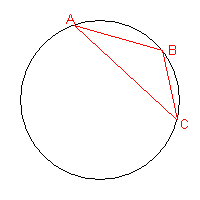If we consider 4 points and trace all the possible chords between them, the circle is divided into 8 regions.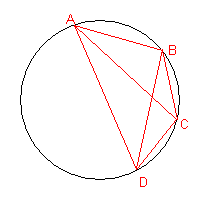If we consider 5 points and trace all the possible chords between them, assuming that no more than two lines intersect at any point inside the circle, the circle is divided into 16 regions.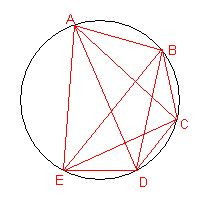Now we might think that, if we add another point, always assuming that no more than two lines intersect at any point inside the circle, the circle will be divided into 32 regions. But it is not true: the regions will be 31.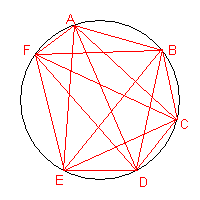As is suggested by D. Acheson in the booklet "1089 and all that" (Oxford University Press, 2002), the number of these regions is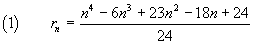The correct sequence can be obtained by observing that, given r1=1,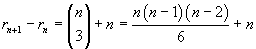Every time we add a new point on the circumference, we get an increase in the number of regions given by the sum of the previous number of triangles with vertices on the circumference, expressed by the binomial coefficient, plus the number of previous points.

For example, when we add the point E,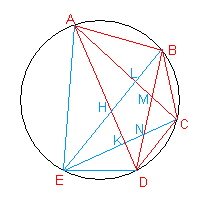we obtain:

• the addition of a circular segment (two circular segments with chords DE and EA instead of the previous segment with chord AD) and of the three triangles EDK, EKH and EHA: then there is an addition of 4 regions;
• the splitting into two parts of the triangles ABM and MCD, then an addition of two regions; the triangle BMC is not split, but in compensation the triangle AMD is split into three parts, with an increase of other two regions; on the whole there is an increase of 4 regions, equal to the number of the pre-existing triangles with vertices on A, B, C and D.

In conclusion, when we add the fifth point we have an increase of 8 regions.

n rn rn-rn-1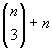1 1
2 2 1 1
3 4 2 2
4 8 4 4
5 16 8 8
6 31 15 15
7 57 26 26
8 99 42 42
9 163 64 64
10 256 93 93

So the equality (1) can be demonstrated by induction. In fact it is true for n = 1 and, taking it true for a given n, we obtain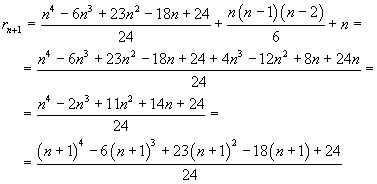So the equality (1) holds for every n.

last revision: May 2018